# Force An example of Force is weight Force

• Slides: 35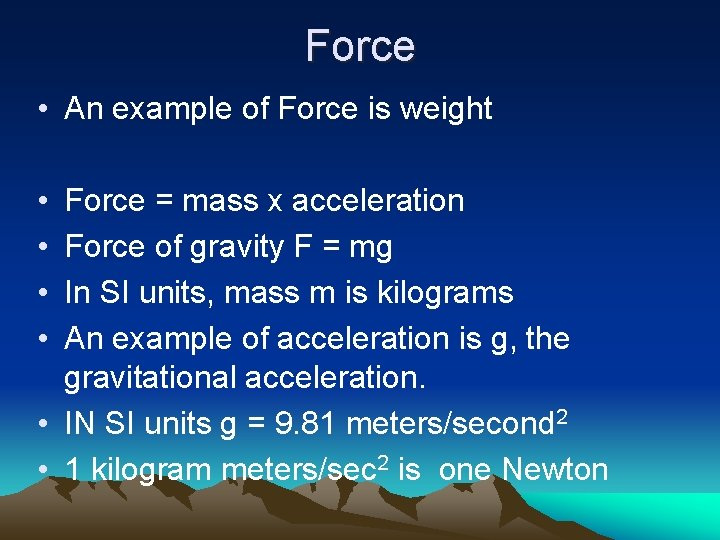Force • An example of Force is weight • • Force = mass x acceleration Force of gravity F = mg In SI units, mass m is kilograms An example of acceleration is g, the gravitational acceleration. • IN SI units g = 9. 81 meters/second 2 • 1 kilogram meters/sec 2 is one NewtonMass Wasting and Hillslopes • Gravity overcomes Friction Steep slope Gd > F F F Moderate slope Gd=F Gd G p 2. Gd = F W Boulder on verge of moving 3. Gd > F. Boulder moves downslope Gp W F Gd Gp W 1. Friction force uphill F is greater than downhill component of gravity. Gd. Boulder is stable Gd Gentle slope Gd < F START HEREMass x gravity = mg = weight “w” Downhill force (white) = mass x gravity x sine of angle a Component of weight along slope (red) Fd = mg sine (a) Shear Force = Fd = w sine (α) dip shear force not shear stress The downhill force, parallel to the incline, Is called the shear force. It is the force that tries to slide the rock down the slope. a w hFriction and Normal Force • Friction Force uphill (yellow) is proportional to Normal Force (green) • Normal Force is perpendicular to the slope. It is the component of weight pressing the rock into the slope. • Increased water pressure between the surfaces lifts the rock, and it will slip at a lower dip angle. dip a w h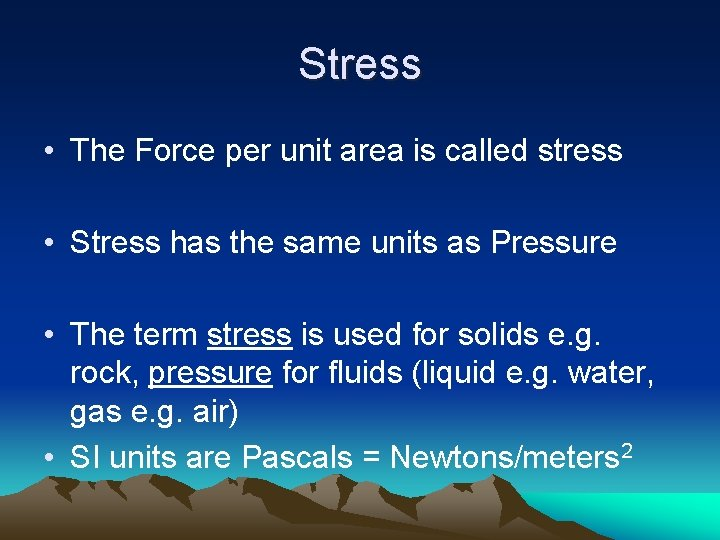Stress • The Force per unit area is called stress • Stress has the same units as Pressure • The term stress is used for solids e. g. rock, pressure for fluids (liquid e. g. water, gas e. g. air) • SI units are Pascals = Newtons/meters 2Stress Sign Conventions • The “standards” • The original sign convention is from Physics and Engineering – For Normal Stress: Divergence lengthens a solid in that direction and is therefore positive – For Shear: Follow the right–hand-rule Look at the x-face, up is positive shear, positive shear turns the element Counter-clockwise All of these conventions vary All positive stresses, both normal and shearStress Sign Conventions • From your text p 47 bottom left” – “The only ingredient left in our description is a sign convention. In physics and engineering, tensile stress is considered positive, and compressive stress negative. In geology, however, it is customary to make compression positive and tension negative, …. ” • Even shear has variable sign conventions • You must be fluent in both conventions, just as you must be fluent in both English and SI units. We will start with the engineering convention.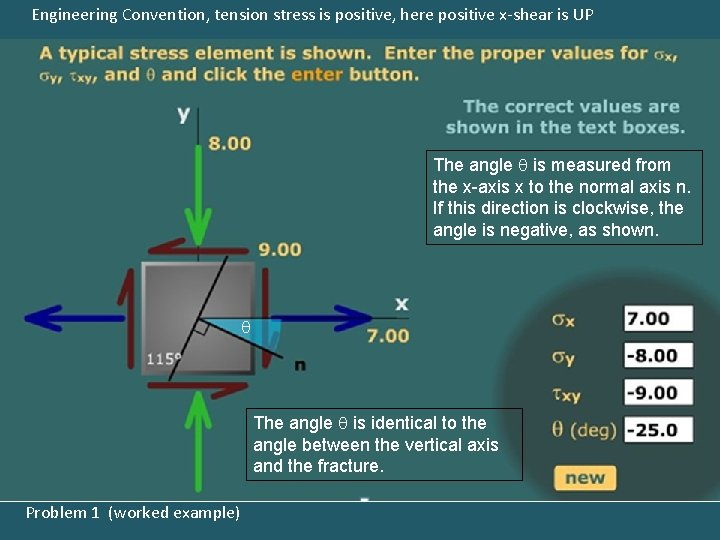Engineering Convention, tension stress is positive, here positive x-shear is UP The angle q is measured from the x-axis x to the normal axis n. If this direction is clockwise, the angle is negative, as shown. q The angle q is identical to the angle between the vertical axis and the fracture. Problem 1 (worked example)Engineering Convention, tension stress is positive Problem 2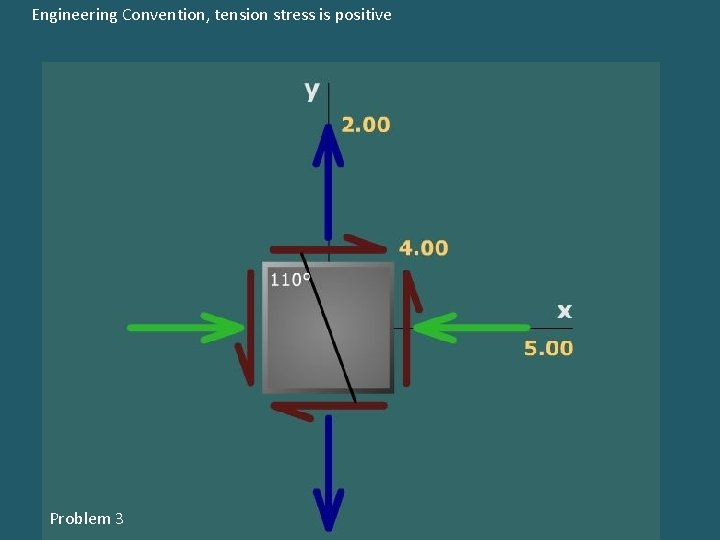Engineering Convention, tension stress is positive Problem 3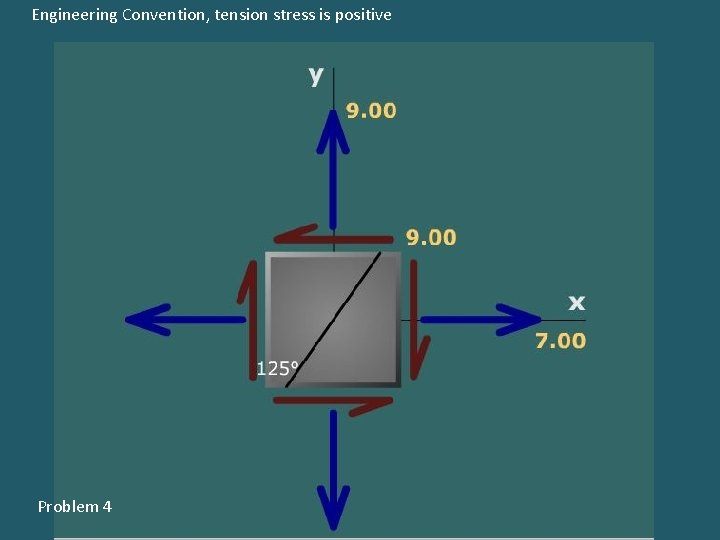Engineering Convention, tension stress is positive Problem 4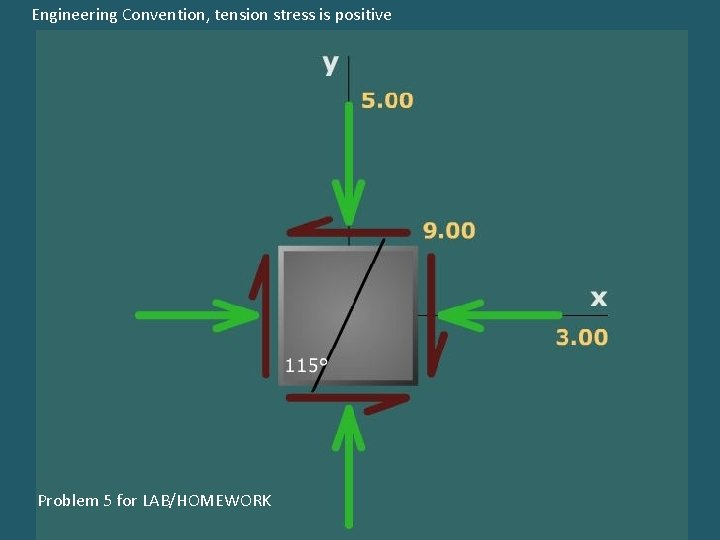Engineering Convention, tension stress is positive Problem 5 for LAB/HOMEWORKEngineering Convention, tension stress is positive Problem 6 for LAB/HOMEWORK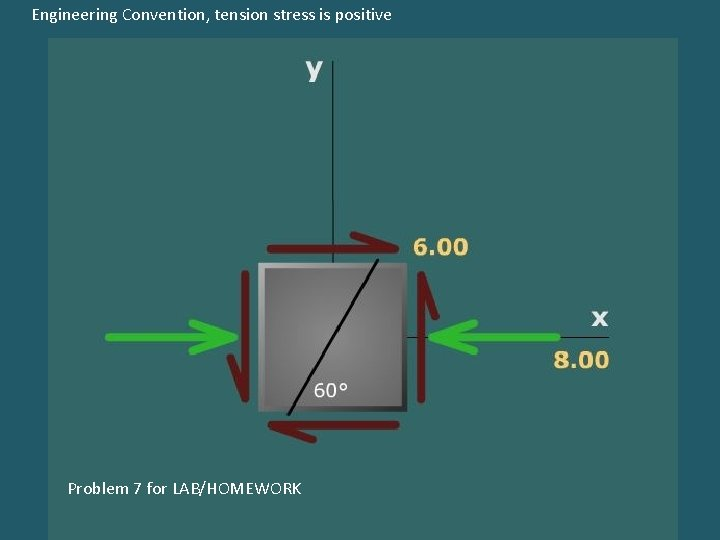Engineering Convention, tension stress is positive Problem 7 for LAB/HOMEWORKEngineering Convention, tension stress is positive Problem 8 for LAB/HOMEWORK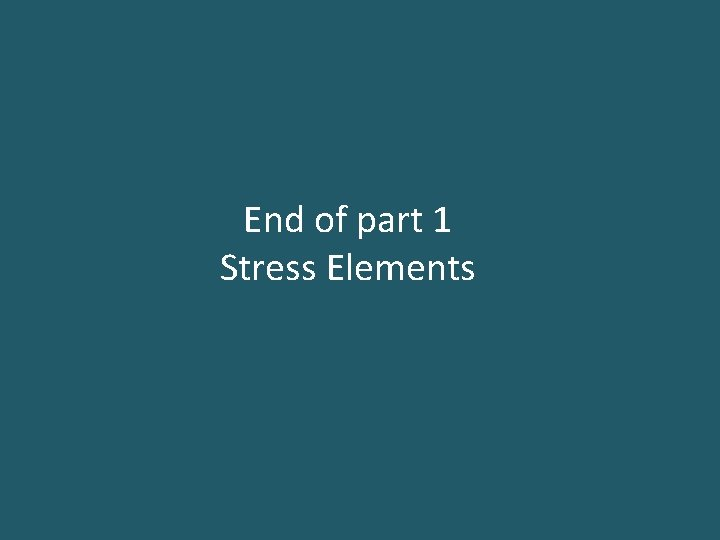End of part 1 Stress Elements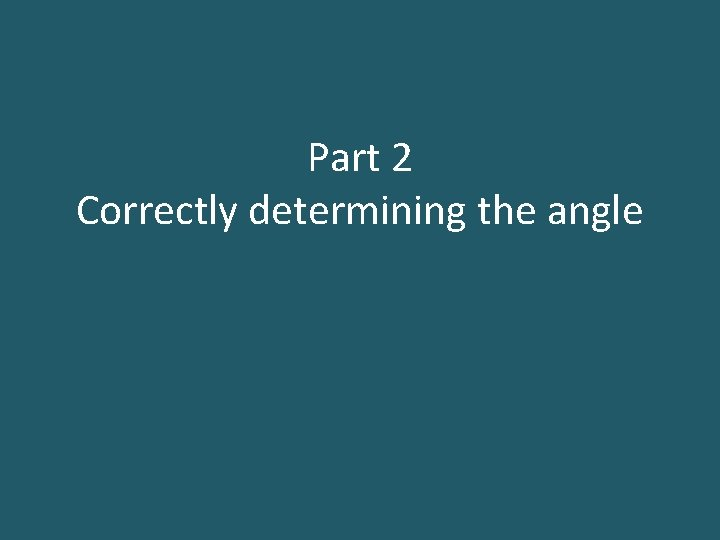Part 2 Correctly determining the angle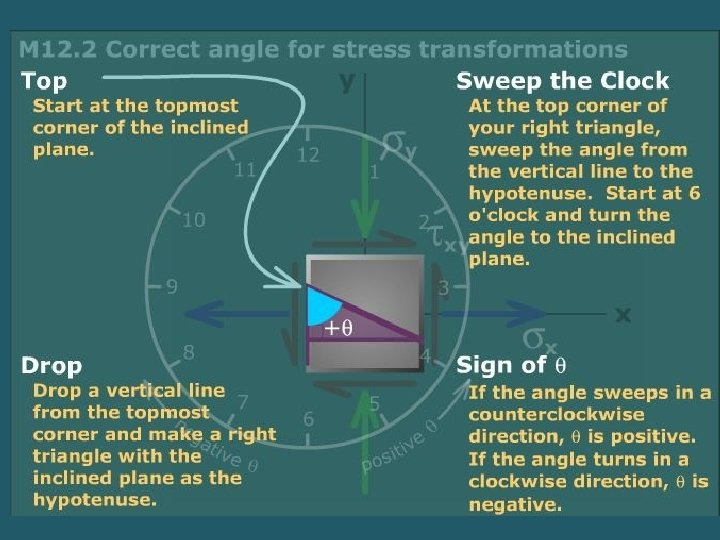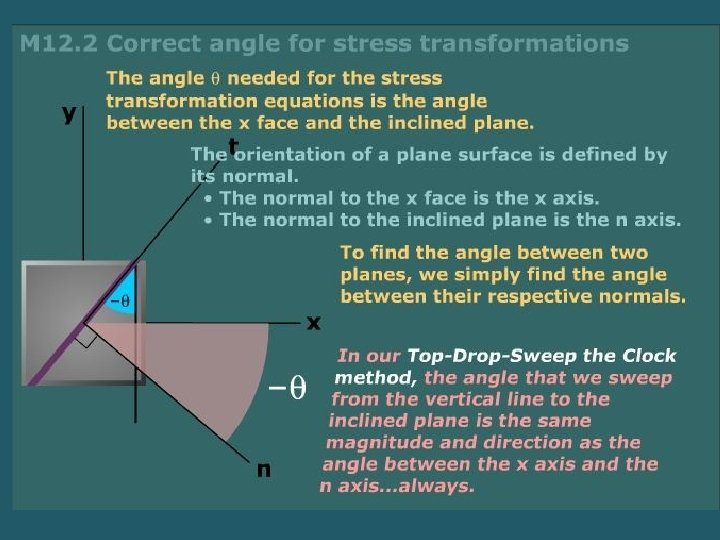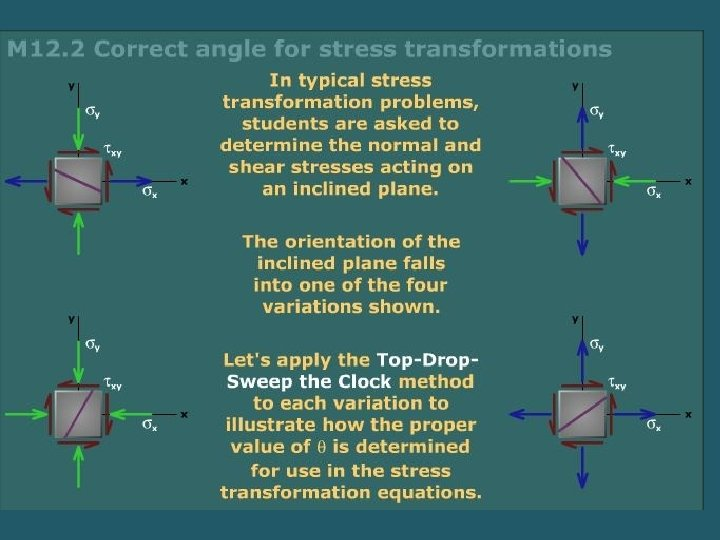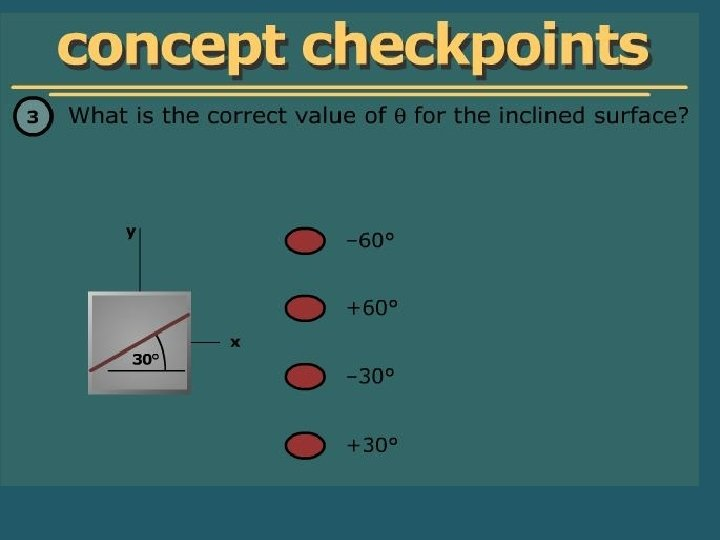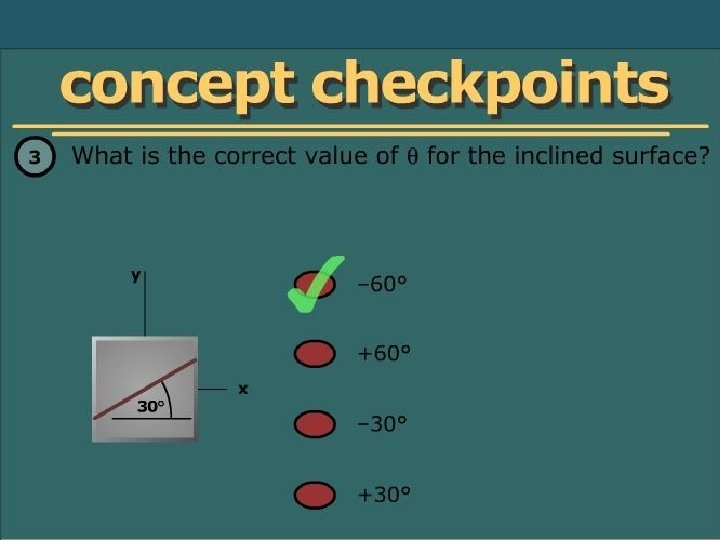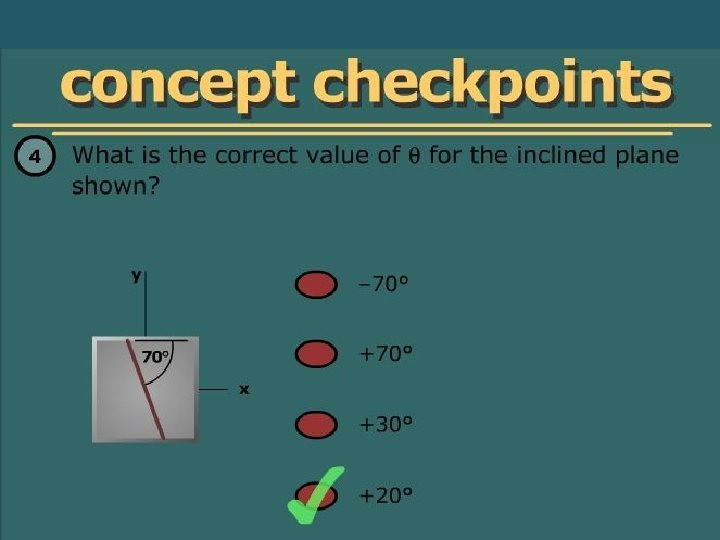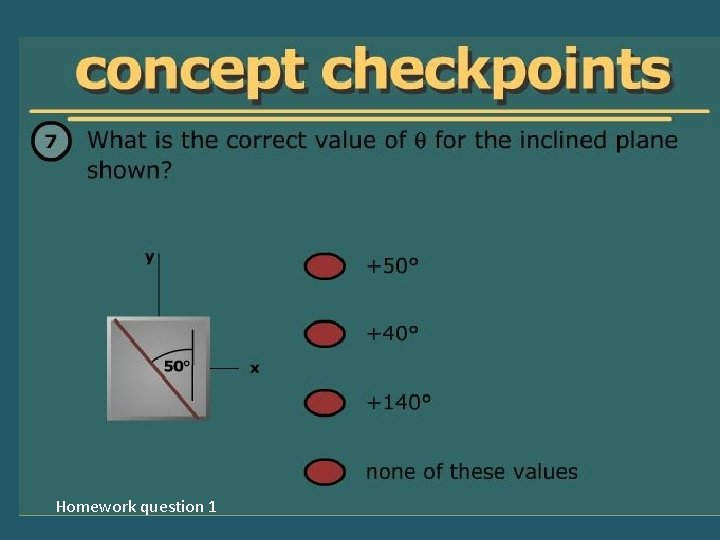Homework question 1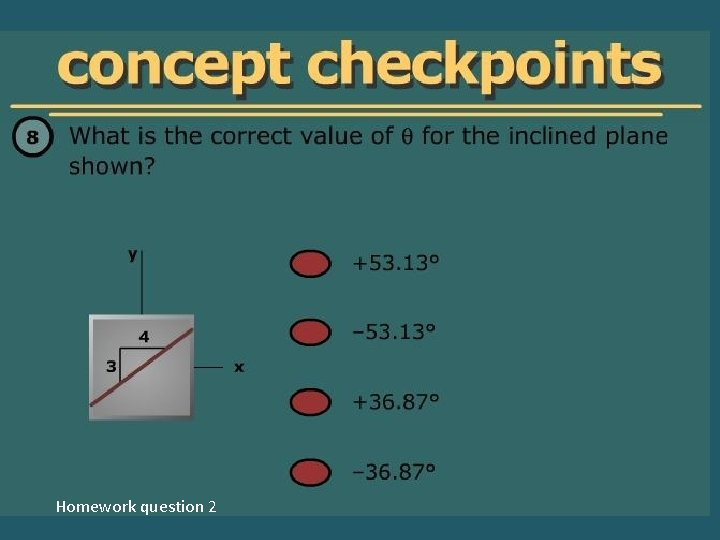Homework question 2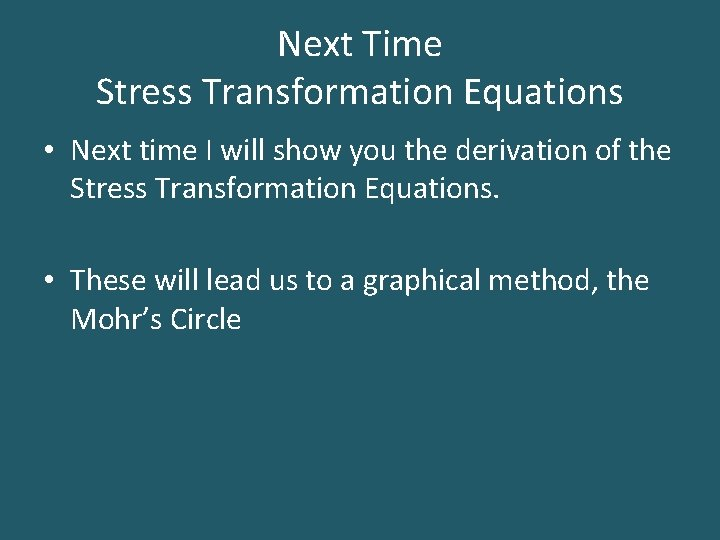Next Time Stress Transformation Equations • Next time I will show you the derivation of the Stress Transformation Equations. • These will lead us to a graphical method, the Mohr’s Circle